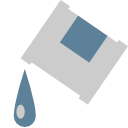# Fill node¶This documentation is for version 1.0 of Fill (fr.inria.Fill).

## Description¶

Add a constant color on the source image where the alpha channel not 0. You can control the blending between the original image and the constant color with the operator and the mix factor.

## Inputs¶

Input Description Optional
Source   No

## Controls¶

Parameter / script name Type Default Function
Convert to Group / convertToGroup Button   Converts this node to a Group: the internal node-graph and the user parameters will become editable
Color / Solid1color Color r: 0 g: 0 b: 0
Operation / Merge1operation Choice over

atop: Ab + B(1 - a) (a.k.a. src-atop)
average: (A + B) / 2
color: SetLum(A, Lum(B))
color-burn: darken B towards A
color-dodge: brighten B towards A
conjoint-over: A + B(1-a)/b, A if a > b
copy: A (a.k.a. src)
difference: abs(A-B) (a.k.a. absminus)
disjoint-over: A+B(1-a)/b, A+B if a+b < 1
divide: A/B, 0 if A < 0 and B < 0
exclusion: A+B-2AB
freeze: 1-sqrt(1-A)/B
from: B-A (a.k.a. subtract)
geometric: 2AB/(A+B)
grain-extract: B - A + 0.5
grain-merge: B + A - 0.5
hard-light: multiply(2*A, B) if A < 0.5, screen(2*A - 1, B) if A > 0.5
hue: SetLum(SetSat(A, Sat(B)), Lum(B))
hypot: sqrt(A*A+B*B)
in: Ab (a.k.a. src-in)
luminosity: SetLum(B, Lum(A))
matte: Aa + B(1-a) (unpremultiplied over)
max: max(A, B) (a.k.a. lighten only)
min: min(A, B) (a.k.a. darken only)
minus: A-B
multiply: AB, A if A < 0 and B < 0
out: A(1-b) (a.k.a. src-out)
over: A+B(1-a) (a.k.a. src-over)
overlay: multiply(A, 2*B) if B < 0.5, screen(A, 2*B - 1) if B > 0.5
pinlight: if B >= 0.5 then max(A, 2*B - 1), min(A, B * 2) else
Mask / Merge1enableMask_Mask Boolean Off
/ Merge1maskChannel_Mask Choice
Invert Mask / Merge1maskInvert Boolean Off
Mix / Merge1mix Double 1